MzNevermore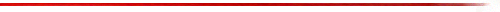### SYNOPSIS

MzNevermore -- Independent control of window size and transform size for spectrogram display.
MzNevermore displays spectrograms in a manner similar to the Raven audio editor from the Cornell Lab of Ornithology.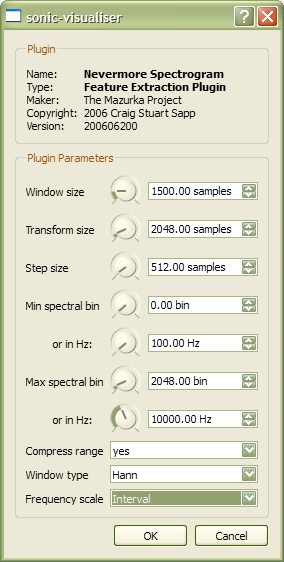### INPUT PARAMETERS

MzNevermore accepts 10 input parameters:

1. Window size
The number of samples of auido data which will be used to calculate the spectrum.
2. Transform size
The size of the windowed audio plus silence padding to reduce vertical pixelization of the spectrum display.
3. Step size
The number of samples to shift in the audio for each spectrogram frame.
4. Min spectral bin
The minimum frequency bin to display in the spectrogram.
5. Min spectral frequency (in Hz)
The minimum frequency in Hz to display in the spectrogram. This value will be used instead of parameter 4 if it is set to a value other than 0.
6. Maximum spectral bin
The maximum frequency bin to display in the spectrogram.
7. Maximum spectral frequency (in Hz)
The maximum frequency in Hz to display in the spectrogram. This value will be used instead of parameter 6 if it is set to a value other than 0.
8. Compress range
Do dynamic range compression on the amplitude values (makes quiet regions more visible).
9. Window type
The analysis window applied to the audio data before the Fourier transform is calculated. Hann is the default value which will work in most cases.
10. Frequency scale
This is the scaling factor for the vertical axis in the spectrogram. The default is "Hertz" which is a linear scale in terms of frequency values which is the most common display format for spectrograms. The "interval" display is a logarithmic scaling which shows a constant width for intervals.

### DESCRIPTION

Below is an idealized spectrogram of a sound which was synthesized from 28 sinewaves, each represented by one of the lines in the following figure. This idealized spectrogram will be used to demonstrate the various parameters which can control the display of the spectrogram in the MzNevermore plugin.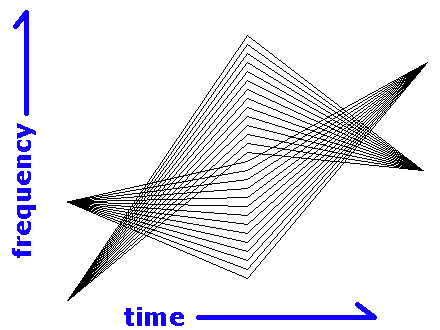```
```

Frequency scale

The frequency scale parameter controls format for the vertical axis of the spectrogram. By default the spectrogram is displayed with "Hertz" linearly on the vertical axis. The other option is "Interval" which displays equivalent intervals equally at any position on the vertical scale.

Since the example ideal spectrogram was used to create the sound on the "Interval" frequency scale, the spectrogram on the right looks more like the ideal spectrogram in this case:

 "Hertz" scaling of frequencies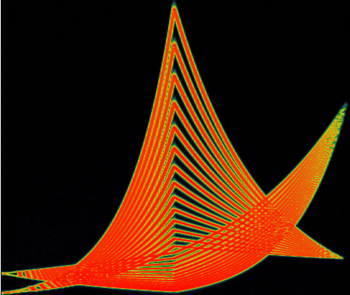"Interval" scaling of frequencies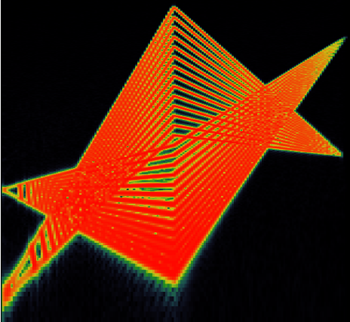"Hertz" scaling is useful when you want to view harmonics as equally spaced lines. "Interval" scalign is useful when you want to view intervales as equally spaced lines.

The difference can perhaps be seen more clearly with a harmonically pitched note as shown in the following figure: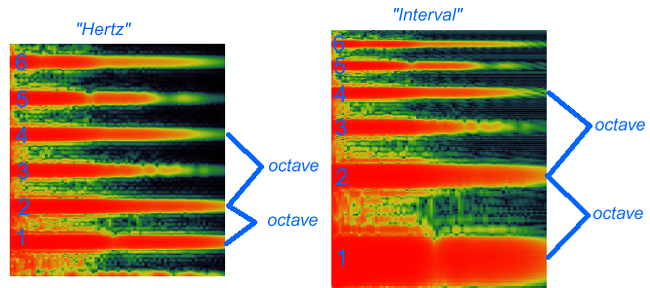The harmonics are labeled from 1 being the fundamental, up to 6 being the 6th harmonic. If the fundamental is 100 Hz, for example, then the 6th harmonic is at 600 Hz. In the "Hertz" vertical scaling, the harmonics are evenly spaced at 100 Hz, 200 Hz, 300 Hz, 400 Hz, 500 Hz, and 600 Hz. In the "Interval" vertical scaling, all interval classes retain their size regardless of their vertical position. For example, two of octaves are marked in the figure above. In the "Interval" scaling, the size of the octaves are equal. In the "Hertz" scaling, the lower octave is 1/2 the size of the octave indicated above it.

Step size

The Step size parameter specifies how many samples to jump between analysis frames in the audio data. A smaller number will give a smoother look to the spectrogram because the spectrogram is updated more often over time. By default, the step size is set to 512 samples. At a 44.1 kHz sampling rate (as used with Compact Disc recordings), that is a time of 512 / 44100 = 11.6 milliseconds. Here is a picture of the center of the spectrogram using a 512 sample step size: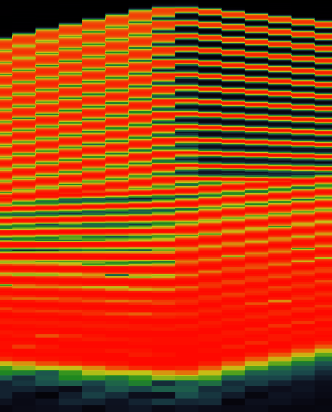Decreasing the step size from 512 to 256 will make the spectrogram display update twice as often: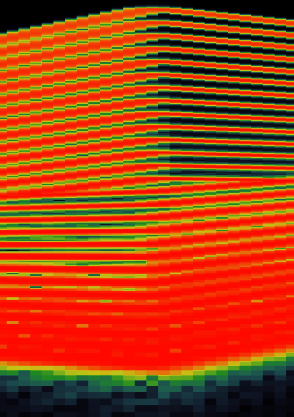Likewise, increasing the step size to 1024 samples (23.2 ms), will increase the quantization on the horizontal time axis: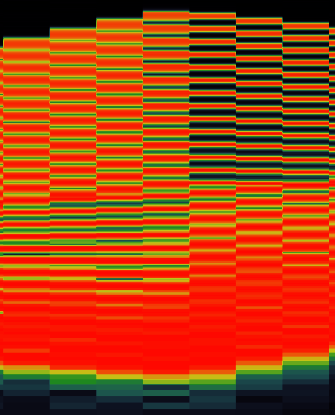If the step size is smaller than the window size, then the audio data between spectrogram frames will overlap.
```
```

Compress range

The Compress range option applies a compression function to the amplitudes (in decibels) in the spectrogram. If this option is selected, a good visual compromise between quiet and loud sounds occurs.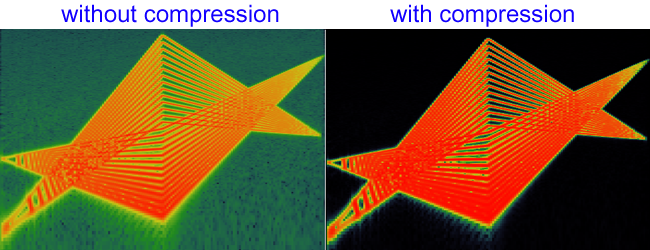The uncompressed range shown on the left contains significant amounts of green in the plot where there is no sound activity. It is displaying the noise floor of the audio file which is at around -96 dB since the file contains 16-bit amplitude values. Since the noise floor is not interesting to see, the compressed amplitude range on the right places the noise floor at the colour black.

The compressed range is best when looking at music which contains a variety of dynamics throughout. However, if you compare the pictures, the compression setting used in this case saturates the peaks near the bottom of the spectrogram, causing a loss of visual frequency discrimination.

```
```

Window size

The Window size parameter controls the frequency resolution ability of the spectrogram. The larger the window size, the more accurately the frequencies can be resolved. However, the larger the window size, the more spectral smearing occurs which decreases the visual sharpness of the display. The following figure demonstrates the trade-off between frequency accuracy and resolution: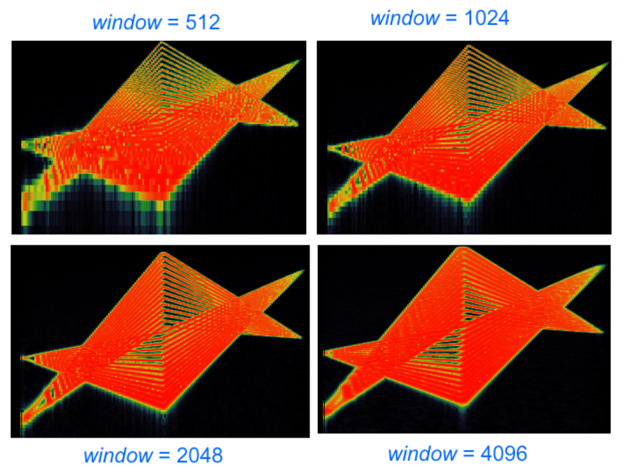For the window size equal to 512, the low frequencies are poorly resolved, since you cannot see the individual harmonic tracks towards the bottom of the spectrogram. As the size of the window increases, the frequency resolution increases, and the lower frequency tracks become clearer.

However, as the window size increases, the harmonic tracks start to smear when they change pitch, and become very sharp when they are at a constant pitch.

If you are analyzing vocal music, violin music or other similar instruments which use vibrato, you must be careful to use a smaller window size so that the frequencies in the vibrato do not smear. For piano and other fixed-pitch instruments, a larger window is more suitable since the smearing would not be as noticeable.

```
```

Transform size

### BUGS

Crashes Sonic Visualiser occasionally for some unknown reason to be examined in the future.

```